edHelper subscribers - Create a new printable

Number of Pages
 Select the number of pages: 1 page 2 pages 3 pages 4 pages

Include an answer key (answer keys will be at the end of the printable)

Sample edHelper.com - Math Review Worksheet

 Name _____________________________Date ___________________
Algebra
State the domain and range for each.
 1 y = (-2x + 2)3 - 31
2.
y =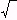-187 - x
 3 y = x2 - 30

Solve each equation by factoring.
4.
 -7x2 - 8x - 9 = -8x2

5.
-4
 23
x2 +
53
 23
x  =
-9
 13
x +
128
 13

 6 24x2 - 34x + 5 = 0

Find the slope and the y-intercept of the line.
 7 6y = -1 + x
 8 3x - y = 3
9.
 x = 4 + 1 y

Factor out the GCF from each polynomial.
 10 20x2 - 24x
 11 -28x3 - 32x2 + 24x
 12 -28x2 - 24x

Complete.
13.   Which represents an irrational number?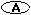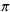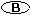-56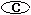36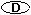-23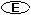34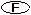0.2
14.   The expression (-8j2g3)2is equivalent to:64j4g6-16j4g5-8j4g564g64j4g5

Complete.
 15 What number, when divided by eight less than the number, is 1 4/25?
 16 Devin has pennies, dimes and quarters. He has a total of \$8.70. There are two times as many pennies as quarters. There are six times as many dimes as quarters. How many of each coin does he have?

Solve each inequality.
17.
 -10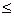2 w

18.
 13 x219

19.
 754 > 29 j

Solve for x.
20.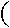-13 18 7+-6 -15 -2x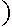--5 8 6=-14 -5 7Sample
This is only a sample worksheet.

edHelper subscribers - Create a new printable

Number of Pages
 Select the number of pages: 1 page 2 pages 3 pages 4 pages